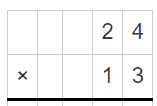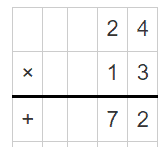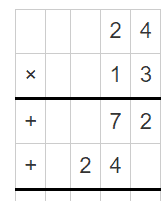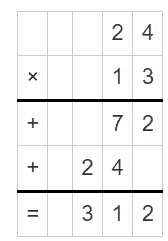# Double Digit Multiplication Worksheets

• ###### Double Digit Multiplication #15

Double digit multiplication worksheets are like your personal math coach. They’re designed to help you understand and practice multiplying numbers that have two digits (like 23 or 84). And trust me, learning this will turn you into a math whiz, making multiplication, and math in general, easier and more fun!

So, let’s delve into the different types of exercises that you’ll find on these worksheets. Each one is designed to be engaging and helpful, guiding you step by step as you explore double-digit multiplication.

Vertical Problems – These are pretty straightforward. You’ll see two numbers with two digits each, one on top of the other, with a multiplication symbol (x) on the side. Your job is to multiply these numbers. This type of exercise helps you practice the process of double-digit multiplication, making you faster and more accurate.

Horizontal Problems – These are similar to vertical problems, but the numbers are written side by side with a multiplication symbol between them. Solving these problems will help you become flexible in how you approach multiplication, allowing you to solve problems no matter how they’re presented.

Fill in the Blank Problems – These problems have a missing number in a multiplication equation that you need to figure out. For example, 14 x __ = 280. These exercises help you think in reverse, enhancing your understanding of multiplication.

Multiple-Step Problems – These exercises contain more than one operation. You might have to add or subtract and multiply in the same problem. These are a bit more challenging, but they’re great for testing your understanding and improving your overall math skills.

So, how can these worksheets and exercises improve your math skills? Well, multiplication is one of the four basic operations in math (along with addition, subtraction, and division). By learning and mastering double-digit multiplication, you’re improving your skills in this fundamental area.

As you practice, you’ll become quicker and more accurate with your multiplication, and this speed and accuracy will help you when you’re working on more complicated math problems. Also, understanding double-digit multiplication will make it easier for you to understand more advanced math topics in the future, like algebra and geometry.

Fill in the blank and multiple-step problems are particularly good for challenging your thinking and improving your understanding of math. They require you to think more deeply about multiplication and how it relates to other operations. This deeper understanding can make you more confident in your math abilities and make math more enjoyable.

In conclusion, double-digit multiplication worksheets are your guide to becoming a multiplication master. They offer a variety of exercises that will help you practice and understand double-digit multiplication in different ways. Through this practice, you’ll improve your speed and accuracy, understand how multiplication is used in real-life situations, and develop valuable problem-solving skills. So next time you see a double-digit multiplication worksheet, remember – it’s not just a piece of paper—it’s an opportunity to grow your math skills and become a more confident mathematician. Happy multiplying!

### How to Solve Double Digit Multiplication Problems

Multiplying two double-digit numbers might seem tricky at first, but with some practice, you’ll get the hang of it. Let’s break down the steps using an example: 24 x 13.

Step 1) Setting up the Problem – Write the problem down vertically, aligning the digits by their place value (ones place, tens place, etc.). Make sure the larger number is on top.Step 2) Multiply the Ones – Start with multiplying the digit in the ones place of the bottom number (in our case, it’s ‘3’ from 13) by each digit of the top number. Multiply ‘3’ by ‘4’ (the digit in the ones place of the number 24) and you get ’12’. Write ‘2’ under the line and carry over the ‘1’ to the tens place.

Step 3) Continue Multiplication – Next, multiply ‘3’ by ‘2’ (the digit in the tens place of the number 24). This equals ‘6’, but don’t forget to add the ‘1’ you carried over. So, ‘6’ + ‘1’ equals ‘7’. Write this down.Step 4) Multiply the Tens – Now move to the digit in the tens place of the bottom number (in our case, it’s ‘1’ from 13). But first, since you’re now working with the tens place, you need to add a zero under your previous answer before you start.

Step 5) Repeat the Multiplication – Now repeat the multiplication process, but this time with the digit in the tens place (‘1’). Multiply ‘1’ by ‘4’, which equals ‘4’, and write this next to the zero. Then multiply ‘1’ by ‘2’, which equals ‘2’. Write this down.Step 6) Add the Results: Now, you add the two results together.So, 24 multiplied by 13 equals 312! That’s how you multiply two double-digit numbers. Remember, practice makes perfect. The more you practice, the better you’ll get at this process.